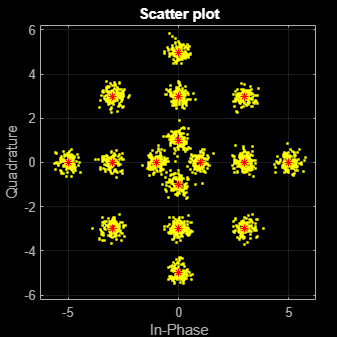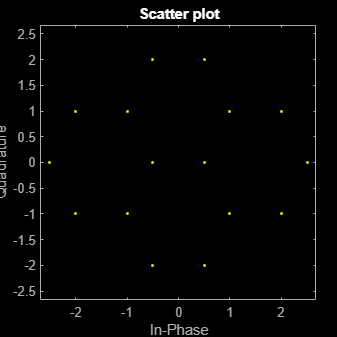# genqammod

## Syntax

``y = genqammod(x,const)``

## Description

example

````y = genqammod(x,const)` returns the complex envelop of the QAM for message signal `x`. Input `const` specifies the signal mapping for the modulation.```

## Examples

collapse all

Transmit and receive data using a nonrectangular 16-ary constellation in the presence of Gaussian noise. Show the scatter plot of the noisy constellation and estimate the symbol error rate (SER) for two different SNRs.

Create a 16-QAM constellation based on the V.29 standard for telephone-line modems.

```c = [-5 -5i 5 5i -3 -3-3i -3i 3-3i 3 3+3i 3i -3+3i -1 -1i 1 1i]; sigpower = pow2db(mean(abs(c).^2)); M = length(c);```

Generate random symbols.

`data = randi([0 M-1],2000,1);`

Modulate the data by using the `genqammod` function. General QAM modulation is necessary because the custom constellation is not rectangular.

`modData = genqammod(data,c);`

Pass the signal through an AWGN channel with a 20 dB SNR.

`rxSig = awgn(modData,20,sigpower);`

Display a scatter plot of the received signal and the reference constellation `c`.

```h = scatterplot(rxSig); hold on scatterplot(c,[],[],'r*',h) grid hold off```Demodulate the received signal by using the `genqamdemod` function. Determine the number of symbol errors and the SER.

```demodData = genqamdemod(rxSig,c); [numErrors,ser] = symerr(data,demodData)```
```numErrors = 4 ```
```ser = 0.0020 ```

Repeat the transmission and demodulation process with an AWGN channel with a 10 dB SNR. Determine the SER for the reduced SNR. As expected, the performance degrades when the SNR is decreased.

```rxSig = awgn(modData,10,sigpower); demodData = genqamdemod(rxSig,c); [numErrors,ser] = symerr(data,demodData)```
```numErrors = 457 ```
```ser = 0.2285 ```

Create the points that describe a hexagonal constellation.

```inphase = [1/2 1 1 1/2 1/2 2 2 5/2]; quadr = [0 1 -1 2 -2 1 -1 0]; inphase = [inphase;-inphase]; inphase = inphase(:); quadr = [quadr;quadr]; quadr = quadr(:); const = inphase + 1i*quadr;```

Plot the constellation.

`h = scatterplot(const);`Generate input data symbols. Modulate the symbols using this constellation.

```x = [3 8 5 10 7]; y = genqammod(x,const);```

Demodulate the modulated signal, `y`.

`z = genqamdemod(y,const);`

Plot the modulated signal in same figure.

```hold on; scatterplot(y,1,0,'ro',h); legend('Constellation','Modulated signal'); hold off;```Determine the number of symbol errors between the demodulated data to the original sequence.

`numErrs = symerr(x,z)`
```numErrs = 0 ```

## Input Arguments

collapse all

Message signal, specified as a scalar, vector, matrix, or 3-D array of numeric values. The message signal must consist of integers from 0 and `length`(`const`)–1. If `x` is a matrix with multiple rows, the function processes the columns independently.

Data Types: `double` | `single` | `fi` | `int8` | `int16` | `uint8` | `uint16`

Signal mapping, specified as a complex vector.

Data Types: `double` | `single` | `fi` | `int8` | `int16` | `uint8` | `uint16`
Complex Number Support: Yes

## Output Arguments

collapse all

Complex envelope, returned as a scalar, vector, matrix, or 3-D array of numeric values. The length of `y` is the same as the length of input `x`.

Data Types: `double` | `single` | `fi` | `int8` | `int16` | `uint8` | `uint16`

## Version History

Introduced before R2006a